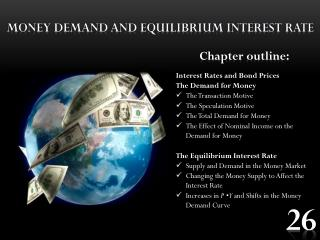# Money demand and equilibrium interest rate - PowerPoint PPT PresentationDownload PresentationMoney demand and equilibrium interest rate

Money demand and equilibrium interest rateDownload Presentation## Money demand and equilibrium interest rate

- - - - - - - - - - - - - - - - - - - - - - - - - - - E N D - - - - - - - - - - - - - - - - - - - - - - - - - - -
##### Presentation Transcript

1. Money demandandequilibriuminterest rate • Chapteroutline: • Interest Rates and Bond Prices • The Demand for Money • The Transaction Motive • The Speculation Motive • The Total Demand for Money • The Effect of Nominal Income on the Demand for Money • The Equilibrium Interest Rate • Supply and Demand in the Money Market • Changing the Money Supply to Affect the Interest Rate • Increases in P • Y and Shifts in the Money Demand Curve 26

2. Interest Rates and Bond Prices interest The fee that borrowers pay to lenders for the use of their funds. Firms and governments borrow funds by issuing bonds, and they pay interest to the lenders that purchase the bonds. When interest rates rise, the prices of existing bonds fall.

3. The Transaction Motive transaction motive The main reason that people hold money—to buy things. nonsynchronization of income and spending The mismatch between the timing of money inflow to the household and the timing of money outflow for household expenses.

4. The Speculation Motive speculation motive One reason for holding bonds instead of money: Because the market price of interest-bearing bonds is inversely related to the interest rate, investors may want to hold bonds when interest rates are high with the hope of selling them when interest rates fall.

5. The Total Demandfor Money The total quantity of money demanded in the economy is the sum: demand for checking account balances +cash by both households and firms. At any given moment, there is a demand for money—for cash and checking account balances. Although households and firms need to hold balances for everyday transactions, their demand has a limit. For both households and firms, the quantity of money demanded at any moment depends on the opportunity cost of holding money, a cost determined by the interest rate.

6. The Total Demandfor Money The quantity of moneydemanded (the amount ofmoney households and firmswant to hold) is a function of theinterest rate.Because the interestrate is the opportunity cost ofholding money balances,increases in the interest ratereduce the quantity of moneythat firms and households wantto hold and decreases in theinterest rate increase the quantityof money that firms andhouseholds want to hold. The Demand Curve for Money Balances

7. The Effect of Nominal Income on the Demand for Money An increase in nominal Aggregate Output (Income) ( P Y ) shifts the money demand curve to the right

8. The Effect of Nominal Income on the Demand for Money The demand for money depends negatively on the interest rate, r, and positively on real income, Y, and the price level, P.

9. The Equilibrium Interest Rate The point at which the quantity of money demanded equals the quantity of money supplied determines the equilibrium interest rate in the economy.

10. Supply and Demand in the Money Market Equilibrium exists in the money market when the supply of money is equal to the demand for money and thus when the supply of bonds is equal to the demand for bonds. At r0 the price of bonds would be bid up(and thus the interest rate down). At r1 the price of bonds would be bid down (and thus the interest rate up).

11. Changing the Money Supply to Affect the Interest Rate An increase in the supply of money from MS0 to MS1 lowers the rate of interest from 7 percent to 4 percent.

12. Increases in P • Y and Shifts in the Money Demand Curve An increase in nominal income (P • Y) shifts the money demand curve from Md0 to Md1, which raises the equilibrium interest rate from 4 percent to 7 percent.